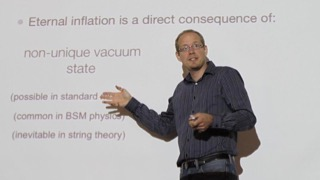Home » Physics and Astronomy Faculty » Research » Theoretical Research

# Theoretical Research

Theoretical Researchers, better known as theoreticians, endeavour to develop conceptual frameworks for understanding the workings of nature that are either revealed or testable by experimentation and measurement. For example, theoretical particle physicists have attempted to reproduce the behaviour of dark matter in galaxies by hypothesizing the existence of a fundamental particle that is self-interacting. Theoretical astrophysicists have devised a magnetohydrodynamic model to explain how a supermassive black hole at the centre of a galaxy is able to eject jets of material accreted from its surroundings. Theoretical biophysicists have made analogies with electrical circuits to understand how neurons in the brain interact.

Picture Name Research Area Research TypeBergevin, Christopher Biological Physics Computational
Experimental
TheoreticalBozorgnia, Nassim Astronomy and Astrophysics
High Energy and Particle Physics
Computational
Theoretical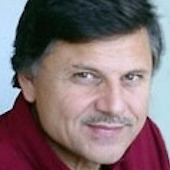Campeanu, Radu Atomic, Molecular and Optical Physics Computational
TheoreticalChen, Yongsheng Planetary Physics Computational
Theoretical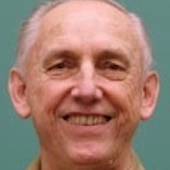Darewych, Jurij Atomic, Molecular and Optical Physics Computational
TheoreticalHall, Patrick Astronomy and Astrophysics Computational
Experimental
Theoretical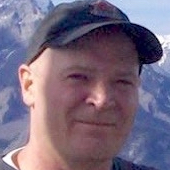Haslam, Michael Applied Mathematics
Atomic, Molecular and Optical Physics
Computational
TheoreticalHessels, Eric Atomic, Molecular and Optical Physics Computational
Experimental
Theoretical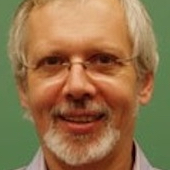Horbatsch, Marko Atomic, Molecular and Optical Physics Computational
TheoreticalJian, Cuiying Chemical and Condensed Matter Physics Computational
TheoreticalJohnson, Matthew Astronomy and Astrophysics
High Energy and Particle Physics
Computational
TheoreticalKirchner, Tom Atomic, Molecular and Optical Physics Computational
Theoretical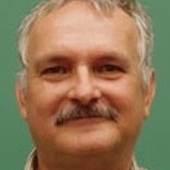Koniuk, Roman High Energy and Particle Physics Computational
Theoretical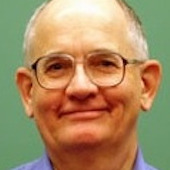Laframboise, James Atomic, Molecular and Optical Physics Computational
Theoretical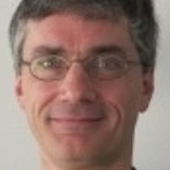Lewis, Randy High Energy and Particle Physics Computational
TheoreticalMadras, Neal Applied Mathematics Computational
Theoretical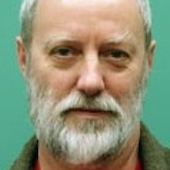Maltman, Kim High Energy and Particle Physics Computational
Theoretical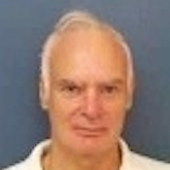McEachran, Robert Atomic, Molecular and Optical Physics Computational
TheoreticalRastgoo, Saeed Astronomy and Astrophysics
High Energy and Particle Physics
Theoretical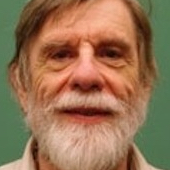Stauffer, Allan Atomic, Molecular and Optical Physics Computational
TheoreticalTulin, Sean Astronomy and Astrophysics
High Energy and Particle Physics
Computational
Theoretical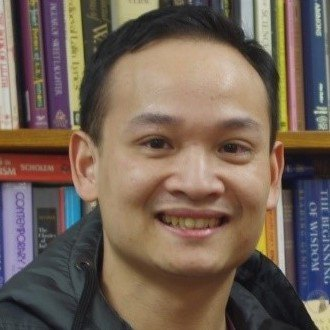Zeng, Toby Physical and Theoretical Chemistry Computational
Theoretical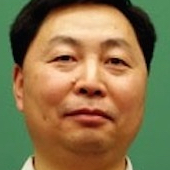Zhu, Zheng Hong Planetary Physics Computational
Experimental
Theoretical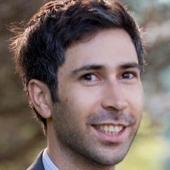Zylberberg, Joel Biological Physics Computational
Theoretical